RS Aggarwal Class 8 Solutions Chapter 5 - Playing With Numbers

RS Aggarwal Class 8 Chapter 5 - Playing With Numbers Solutions Free PDF

RS Aggarwal class 8 solutions chapter 5 playing with Number is provided here. In this chapter,
the students will learn how to find the missing number in a sum or in a subtraction problem. To answer all the questions asked from this chapter and to understand the chapter students are advised to solve questions from RS Aggarwal for class 8.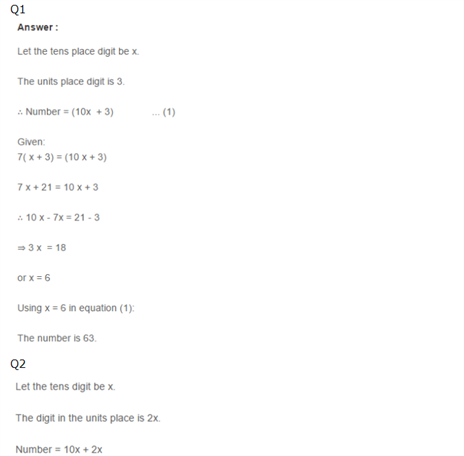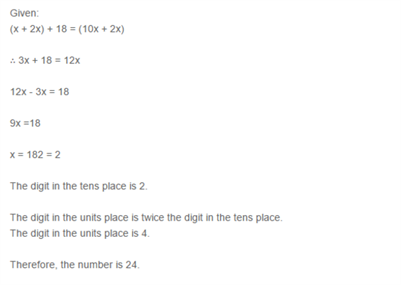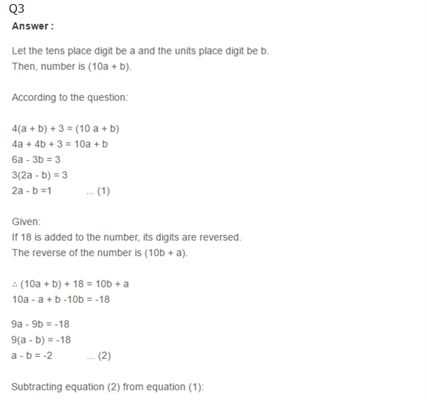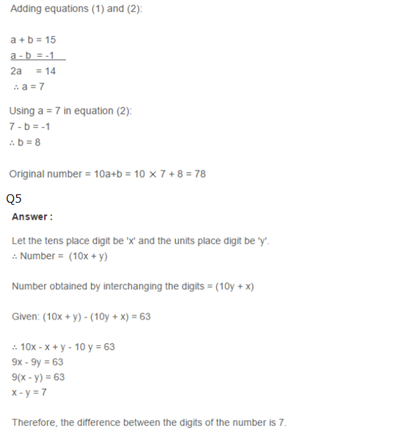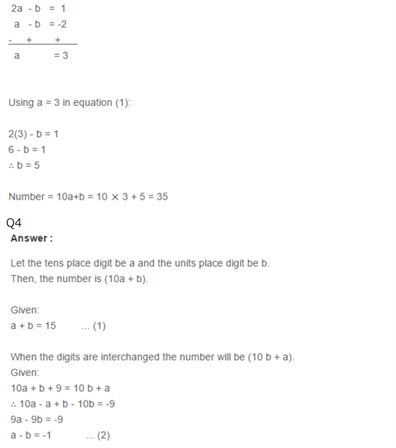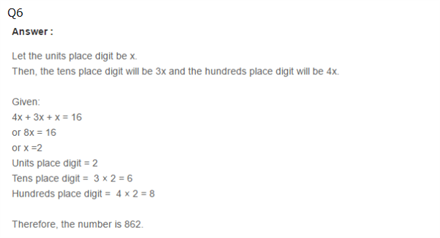Practise This Question

Who exerts the maximum pressure?